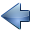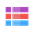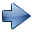9.4 关联容器：QHash、QMultiHash和QSet

9.4.1 单哈希映射 QHash

QHash 是基于哈希表实现的字典结构，存储 key-value 数据对。哈希表可以将任意长度的数据根据特定算法计算固定长度的“精简版”数值，就是数据条目的哈希值。简要来说，对于 key-value 数据对，我们根据 key 的数值计算得到哈希值作为数据存储空间的序号，在该序号位置保存 value，那么我们就可以快速地根据 key 访问到 value 数值。实际中的哈希表结构更复杂，因为要考虑哈希值碰撞的问题，本节就不讨论了。上一节 QMap 利用红黑树实现，读取一个元素的平均效率是 O(logN) ，N 是元素总数。而通过 QHash 读取一个元素，只需要计算 key 对应的哈希值，不需要遍历查找树形结构或数组结构，哈希表的访问效率平均为 O(1) 。
QHash 的优点是通常情况下访问元素效率极高，但是也有缺点，元素排列是无序的，元素排列不按照数值大小或字典序，是按照哈希算法计算的值进行排列，这些哈希值都是杂乱无章的数 值。如果需要有序的数据结构，那么 QMap 就更实用。

QHash 与 QMap 的大部分功能函数名一样，存储的数据也都必须是可赋值类型。但 QHash 与 QMap 有三个重要区别，列举如下：
① QHash 拥有更快的元素查询效率，平均为 O(1)，QMap 平均为 O(logN) 。
② 在 QHash 对象里进行元素遍历时，元素排列杂乱无章，而 QMap 总是按照 key 的排序排列。
③ QHash 的 key 类型必须提供  operator==() 运算符函数和  qHash(Key)  全局函数，而 QMap 的 key 类型则必须提供 operator<() 运算符函数。

（1）构造函数
QHash()  //默认构造函数
QHash(std::initializer_list<std::pair<Key, T> > list)
QHash(const QHash<Key, T> & other)
QHash(QHash<Key, T> && other)
~QHash()  //析构函数

QHash<QString ,int> nameAge = { {"Alice", 20},
{"Bob", 22},
{"Kal", 19} };
qDebug()<<nameAge;
QHash<QString ,int> nameAge2 = std::move( nameAge );
qDebug()<<nameAge;
qDebug()<<nameAge2;

QHash(("Alice", 20)("Kal", 19)("Bob", 22))
QHash()
QHash(("Alice", 20)("Kal", 19)("Bob", 22))

（2）添加函数
对于单哈希映射，通常使用下面的添加函数：
iterator    insert(const Key & key, const T & value)
insert() 函数负责添加新元素，或者替换旧的同 key 元素，如果哈希映射之前没有 key 键元素，那么为哈希映射新增一个元素，键为 key，映射的值为 value；如果之前存在键为 key 的元素，那么替换该元素的映射值为新的 value。QHash 本身是支持多哈希映射功能的，但一般不建议这样用。对用于多哈希映射场景 QHash  对象，如果存在多个键为 key 的元素，那么 insert() 函数替换最近添加的同 key 元素。

iterator    insertMulti(const Key & key, const T & value)
insertMulti() 总是为哈希映射添加新元素，而不管之前有无同 key 元素，也不管是否有同 key-value 对的元素。

（3）移除和删除函数

void    clear()
如果希望根据键 key 来删除元素，使用下面函数：
int    remove(const Key & key)
remove() 函数删除所有键为 key 的元素，返回值是删除的匹配元素个数，如果没有匹配元素，那么返回 0。

T    take(const Key & key)

iterator    erase(iterator pos)
erase() 返回删除元素之后下一个元素的迭代器位置，有可能是迭代器末尾位置。

（4）访问和查询函数

int    count() const
int    size() const

int    count(const Key & key) const
QHash 对象内部使用哈希表，对于哈希表的容量，可以进行查询、扩容、以及缩减空余等操作：
int    capacity() const      //查询哈希表容量
void    reserve(int size)   //提前预留好参数里指定个数的哈希表，扩容操作
void    squeeze()             //缩减哈希表未使用的冗余空间

bool    isEmpty() const // Qt 风格命名，检查哈希映射对象是否为空
bool    empty() const    // STL 风格命名，检查哈希映射对象是否为空

bool    contains(const Key & key) const

const_iterator    constFind(const Key & key) const  // Qt  风格命名，查找匹配 key 元素的只读迭代器
const_iterator    find(const Key & key) const // STL 风格命名，查找匹配 key 元素的只读迭代器
iterator    find(const Key & key) //查找匹配 key 元素的读写迭代器

const T    value(const Key & key) const
const T    value(const Key & key, const T & defaultValue) const

QList<T>    values() const

QList<T>    values(const Key & key) const

const Key    key(const T & value) const
const Key    key(const T & value, const Key & defaultKey) const

QList<Key>    keys() const  //多个相同 key  算做多个

QList<Key>    uniqueKeys() const  //多个相同 key 只算一个

QList<Key>    keys(const T & value) const

（5）交换函数和合并函数
将 QHash 对象自身与另一个 QHash 对象（key 类型、value 类型要一样）的内容全部交换，使用下面成员函数：
void    swap(QHash<Key, T> & other)

QHash<Key, T> &    unite(const QHash<Key, T> & other)
unite()  函数总是添加新元素，同样 key 的元素只会增加，不会替换，所以很可能会形成多重映射。

（6）运算符函数

QHash<QString, int> h1;
h1["Ali"] = 99;
QHash<QString, int> h2;
h2["Bob"] = 88;
h2["Kal"] = 77;

 运算符函数 举 例 描述 bool operator!=(const QHash & other) const h1 != h2; 两个哈希对象的元素不一样，不等号判断结果为 true。 bool operator==(const QHash & other) const h1 == h2; 两个哈希对象的元素不一样，等于号判断结果为 false。 QHash & operator=(const QHash & other) h1 = h2; 将 h2 所有元素复制给 h1，执行后二者相等。 QHash & operator=(QHash && other) h1 = std::move(h2); 将 h2 中所有元素移动给h1，h2自己清空。 T & operator[](const Key & key) h2["Kal"] = 18; 修改了 "Kal" 对应的value值。 const T operator[](const Key & key) const qDebug()<< h2["Kal"]; 打印 "Kal" 对应的常量值。

两个哈希对象相等只需要二者拥有的键值对一样就行了，如果键值对的前后顺序不同，那么不会影响等于号判断。比如两个哈希对象，第一个哈希 对象三个元素 在内存中的存储序列为：
("Bob", 98), ("Ali", 97), ("Cal", 99)

("Ali", 97), ("Bob", 98), ("Cal", 99)

（7）迭代器函数

class    const_iterator   //STL风格只读迭代器
class    iterator             //STL风格读写迭代器
typedef    ConstIterator  //Qt 风格只读迭代器
typedef    Iterator          //Qt风格读写迭代器
STL 风格迭代器使用示范：
QHash<QString, int>::const_iterator i = hash.constBegin();
while (i != hash.constEnd()) {
cout << i.key() << ": " << i.value() << endl;
++i;
}
QHash 对象中的元素排列是无序的，是通过内部哈希函数计算元素排列位置，元素前后序列难以预知。

iterator    begin()     //指向头部的读写迭代器
const_iterator    begin() const            //指向头部的只读迭代器
const_iterator    cbegin() const          //指向头部的只读迭代器
const_iterator    constBegin() const  //指向头部的只读迭代器，Qt风格
iterator    end()       //指向尾部后面假想元素的读写迭代器
const_iterator    end() const             //指向尾部后面假想元素的只读迭代器
const_iterator    cend() const           //指向尾部后面假想元素的只读迭代器
const_iterator    constEnd() const    //指向尾部后面假想元素的只读迭代器，Qt风格

（8）默认支持哈希的类型

uint    qHash(const QString & key, uint seed = 0)  //计算字符串的哈希值
uint    qHash(const T * key, uint seed = 0)              //计算任意数据指针的哈希值
uint    qHashBits(const void * p, size_t len, uint seed = 0)  //辅助函数，用于编写新类型的 qHash() 函数，可以根据任意内存块生成哈希值

（9）其他内容
QHash 模板类也支持串行化输出和输入，通过以下函数实现：
QDataStream &    operator<<(QDataStream & out, const QHash<Key, T> & hash) //输出到数据流
QDataStream &    operator>>(QDataStream & in, QHash<Key, T> & hash)  //从数据流输入

① 不能使用 hash[key] 这种形式查找哈希对象里是否包含键 key 的元素，因为 operator[](const Key & key) 函数在找不到 key 键元素时，自动调用 value 类默认构造函数为哈希对象添加新的 key-value 哈希映射元素。

② 一般不建议使用 QHash 的 insertMulti() 和 unite() 函数进行多重哈希映射添加或哈希映射合并，Qt 单独提供了 QMultiHash表示多重哈希映射，在程序中尽量让 QHash 保持一对一映射，避免代码的误解。

QHash 类的使用方法与 QMap 非常类似，因此本小节留一个练习，就是将之前 9.3.1 小节 nameage 示例中 QMap 内容改用 QHash 实现。

①项目名称 persontoid，创建路径 D:\QtProjects\ch09，点击下一步；
②套件选择里面选择全部套件，点击下一步；
③基类选择 QWidget，点击下一步；
④项目管理不修改，点击完成。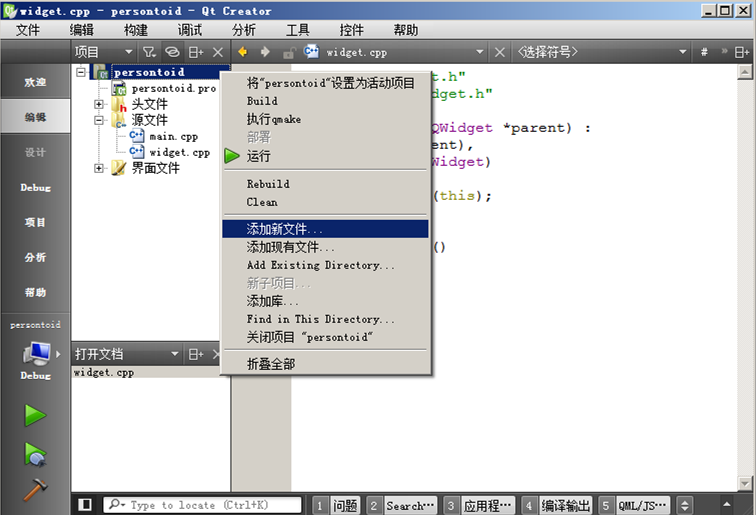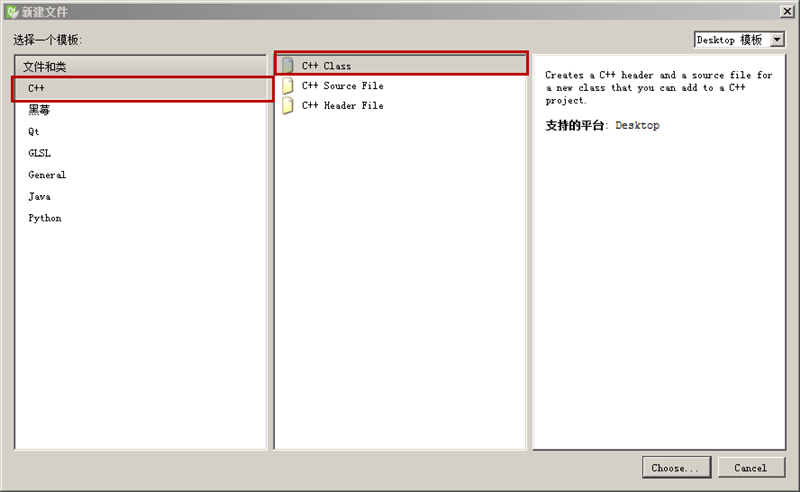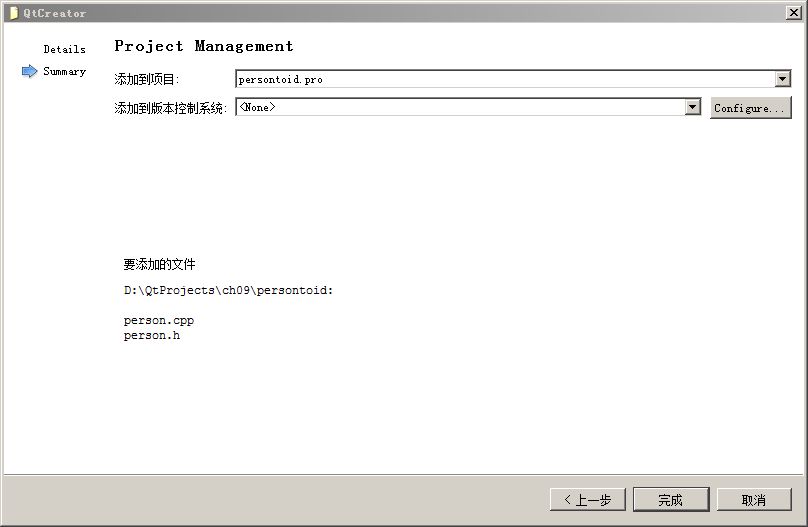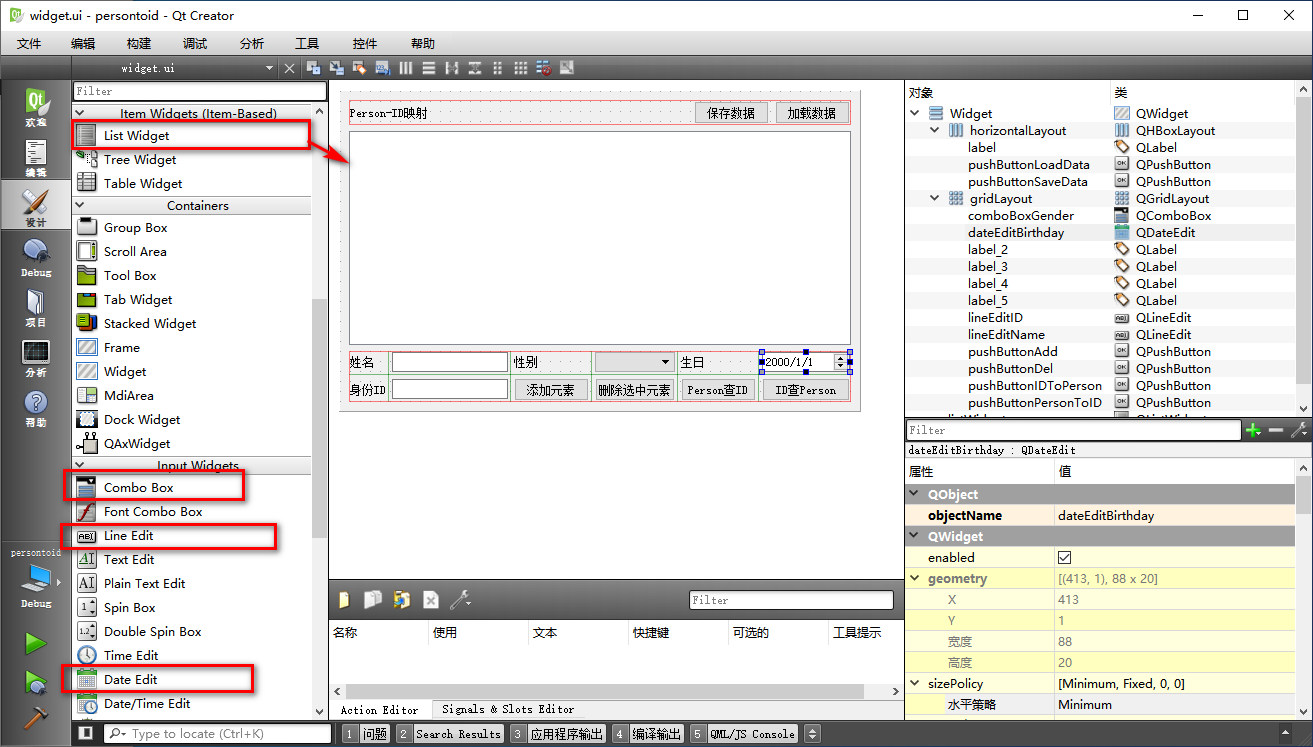#ifndef PERSON_H
`#define PERSON_H`

`#include <QHash>`
`#include <QMap>`
`#include <QString>`
`#include <QDateTime>`
`#include <QDataStream>`

`class Person`
`{`
`public:`
`    //带参数的构造函数`
`    Person(QString strName, QString strGender, QDate birthDay);`

`    //数据库容器对象只能存储可赋值数据，key和value都要求可赋值`
`    //不带参数的默认构造函数，可赋值类型要求 1`
`    Person();`
`    Person(const Person &other);    //复制构造函数，可赋值类型要求2`
`    Person &operator =(const Person &other);//赋值号，可赋值类型要求3`
`    ~Person();`

`    //姓名、性别、出生日期，三个成员变量读写函数，只读函数要加 const 修饰`
`    inline QString name() const`
`    {`
`        return m_name;`
`    }`
`    inline void setName(const QString &strName)`
`    {`
`        m_name = strName;`
`    }`
`    inline QString gender() const`
`    {`
`        return m_gender;`
`    }`
`    inline void setGender(const QString &strGender)`
`    {`
`        m_gender = strGender;`
`    }`
`    inline QDate birthday() const`
`    {`
`        return m_birthday;`
`    }`
`    inline void setBirthday(const QDate &bday)`
`    {`
`        m_birthday = bday;`
`    }`

`protected:`
`    //姓名、性别、出生日期`
`    QString m_name;`
`    QString m_gender;`
`    QDate m_birthday;`
`};`

`//哈希对于key类型额外要求 1，全局的等于号比较函数`
`bool operator ==(const Person &p1, const Person &p2);`

`//哈希对于key类型额外要求 2，全局的哈希函数`
`uint qHash(const Person &key, uint seed=0);`

`//输入输出串行化要求，全局的串行化函数`
`QDataStream &operator <<(QDataStream &out, const Person &pout);`
`QDataStream &operator >>(QDataStream &in, Person &pin);`

`//补充说明，如果用于 QMap，那么实现全局的小于号比较函数，该函数还可以用于 qSort()排序函数`
`bool operator <(const Person &p1, const Person &p2);`

`#endif // PERSON_H`

#include "person.h"

`//带参数的构造函数`
`Person::Person(QString strName, QString strGender, QDate birthDay)`
`{`
`    m_name = strName;`
`    m_gender = strGender;`
`    m_birthday = birthDay;`
`}`
`//默认构造函数，可赋值类型要求 1`
`Person::Person()`
`{`
`    m_name = "";`
`    m_gender = "";`
`    m_birthday = QDate(2000, 1, 1);`
`}`
`//复制构造函数，可赋值类型要求2`
`Person::Person(const Person &other)`
`{`
`    m_name = other.m_name;`
`    m_gender = other.m_gender;`
`    m_birthday = other.m_birthday;`
`}`
`//赋值运算符，可赋值类型要求3`
`Person &Person::operator =(const Person &other)`
`{`
`    m_name = other.m_name;`
`    m_gender = other.m_gender;`
`    m_birthday = other.m_birthday;`
`    //返回对象`
`    return *this;`
`}`
`//默认析构函数`
`Person::~Person()`
`{`

`}`

//哈希对于key类型额外要求 1，全局的等于号比较函数
`bool operator ==(const Person &p1, const Person &p2)`
`{`
`    bool bRet = false; //默认不等`
`    if(  (p1.name() == p2.name())`
`      && (p1.gender() == p2.gender())`
`      && (p1.birthday() == p2.birthday()) )`
`    {`
`        bRet = true; //三个成员都相等`
`    }`
`    //返回`
`    return bRet;`
`}`
`//哈希对于key类型额外要求 2，全局的哈希函数`
`uint qHash(const Person &key, uint seed)`
`{`
`    uint nRet;`
`    nRet = qHash(key.name(), seed)`
`            ^ qHash(key.gender(), seed)`
`            ^ qHash(key.gender(), seed);`
`    return nRet;`
`}`
==() 比较运算符函数仅在三个成员值都相等的情况下返回 true ，其他情况均为 false。

//输入输出串行化要求，全局的串行化函数
`QDataStream &operator <<(QDataStream &out, const Person &pout)`
`{`
`    out<<pout.name()<<pout.gender()<<pout.birthday();`
`    return out;`
`}`

`QDataStream &operator >>(QDataStream &in, Person &pin)`
`{`
`    //定义三个变量接受输入数据`
`    QString strName;`
`    QString strGender;`
`    QDate birthday;`
`    in>>strName>>strGender>>birthday;`
`    //设置成员变量`
`    pin.setName( strName );`
`    pin.setGender( strGender );`
`    pin.setBirthday( birthday );`
`    return in;`
`}`

person.cpp 最后末尾是补充的 <() 运算符函数，可以用于支持 QMap key 类型，或者用于 qSort() 排序函数：
//补充说明，如果用于 QMap，那么实现全局的小于号比较函数，该函数还可以用于 qSort()排序函数
`bool operator <(const Person &p1, const Person &p2)`
`{`
`    //按照姓名字典序大小比较`
`    return ( p1.name() < p2.name() );`
`}`
<()  比较函数用的是使用 name() 函数返回值比较，按字典序排列。
Person 类及其相关代码可以支持 QHash、QMap 的 key 类型，方便使用。以后使用容器遇到需要自定义的 key 类型，可以参照上面代码实现。

#ifndef WIDGET_H
`#define WIDGET_H`

`#include <QWidget>`
`#include "person.h"`

`namespace Ui {`
`class Widget;`
`}`

`class Widget : public QWidget`
`{`
`    Q_OBJECT`

`public:`
`    explicit Widget(QWidget *parent = 0);`
`    ~Widget();`

`    //显示更新后的数据`
`    void UpdateDataShow();`

`private slots:`
`    void on_pushButtonAdd_clicked();`

`    void on_pushButtonDel_clicked();`

`    void on_pushButtonPersonToID_clicked();`

`    void on_pushButtonIDToPerson_clicked();`

`    void on_pushButtonSaveData_clicked();`

`    void on_pushButtonLoadData_clicked();`

`private:`
`    Ui::Widget *ui;`

`    //定义容器`
`    QHash<Person, quint64> m_data;`
`};`

`#endif // WIDGET_H`
widget.h 添加了 "person.h" 头文件包含。在类声明里面我们添加了一个 UpdateDataShow() 函数，专门用于在数据容器内容变化时，将内容显示到界面的列表控件中，然后声明了使用 Person 类型为 key，使用 quint64 类型为 value 的哈希映射类型对象 m_data 。其他函数是自动生成的，有 6 个按钮对应的槽函数。

#include "widget.h"
`#include "ui_widget.h"`
`#include <QMessageBox>`
`#include <QDebug>`
`#include <QFile>`
`#include <QFileDialog>`
`#include <QDataStream>`

`Widget::Widget(QWidget *parent) :`
`    QWidget(parent),`
`    ui(new Ui::Widget)`
`{`
`    ui->setupUi(this);`
`    //添加性别`
`    ui->comboBoxGender->addItem( tr("男") );`
`    ui->comboBoxGender->addItem( tr("女") );`
`    //日历控件编辑时，点击右边小按钮弹出日历表`
`    ui->dateEditBirthday->setCalendarPopup( true );`

`}`

`Widget::~Widget()`
`{`
`    delete ui;`
`}`

//显示更新后的数据
`void Widget::UpdateDataShow()`
`{`
`    //清空旧的显示`
`    ui->listWidget->clear();`
`    //获取key列表`
`    QList<Person> listPersons = m_data.keys();`
`    //个数`
`    int nCount = listPersons.count();`
`    for (int i=0; i<nCount; i++)`
`    {`
`        //字符串`
`        QString strLine;`
`        strLine = listPersons[i].name() + tr("\t")`
`                + listPersons[i].gender() + tr("\t")`
`                + listPersons[i].birthday().toString( "yyyy-MM-dd" );`
`        //ID`
`        quint64 theID = m_data[ listPersons[i] ] ;`
`        strLine += tr("\t%1").arg( theID );`
`        //添加到列表显示`
`        ui->listWidget->addItem( strLine );`
`    }`
`    //处理完成`
`}`

//添加元素
`void Widget::on_pushButtonAdd_clicked()`
`{`
`    //检查姓名和ID`
`    QString strName = ui->lineEditName->text().trimmed();`
`    if( strName.isEmpty() )`
`    {`
`        QMessageBox::warning(this, tr("检查姓名"), tr("姓名为空，不能添加！"));`
`        return;`
`    }`
`    quint64 nID;`
`    bool bOK = false; //用于检查转换是否出错`
`    nID = ui->lineEditID->text().toULongLong( &bOK, 10 );`
`    if( ! bOK )`
`    {`
`        QMessageBox::warning(this, tr("ID检查"), tr("请输入合法数字ID！"));`
`        return;`
`    }`
`    //性别生日`
`    QString strGender = ui->comboBoxGender->currentText();`
`    QDate birthday = ui->dateEditBirthday->date();`
`    //构造 Person`
`    Person pkey( strName, strGender, birthday );`
`    //添加元素`
`    m_data[ pkey ] = nID;`
`    //更新显示`
`    UpdateDataShow();`
`}`

m_data[ pkey ] = nID;

//删除选中元素
`void Widget::on_pushButtonDel_clicked()`
`{`
`    //当前条目`
`    QListWidgetItem *pCurItem = ui->listWidget->currentItem();`
`    if( NULL == pCurItem )`
`    {`
`        return;`
`    }`
`    if( ! (pCurItem->isSelected()) )`
`    {`
`        //未被选中，不删除`
`        qDebug()<<tr("未选中条目，不做删除操作");`
`        return;`
`    }`
`    //将文本转为变量`
`    QString strAll = pCurItem->text();`
`    QStringList listParas = strAll.split( "\t" );`
`    qDebug()<<tr("删除元素：")<<listParas;`
`    // 0号是名字，1号是性别，2号是生日，3号是ID`
`    QDate birthday = QDate::fromString( listParas, "yyyy-MM-dd" );`
`    Person pkey( listParas, listParas, birthday );`
`    //删除变量元素`
`    m_data.remove( pkey );`
`    //更新显示`
`    UpdateDataShow();`
`}`

//Person查询ID
`void Widget::on_pushButtonPersonToID_clicked()`
`{`
`    //检查姓名`
`    QString strName = ui->lineEditName->text().trimmed();`
`    if( strName.isEmpty() )`
`    {`
`        QMessageBox::warning(this, tr("检查姓名"), tr("姓名为空，不能添加！"));`
`        return;`
`    }`
`    //性别、生日`
`    QString strGender = ui->comboBoxGender->currentText();`
`    QDate birthday = ui->dateEditBirthday->date();`
`    //对象`
`    Person pkey( strName, strGender, birthday );`

`    //一定要用 contains 函数查询 key 是否存在`
`    if( m_data.contains( pkey ) )`
`    {`
`        quint64 nID = m_data[ pkey ];`
`        QString strInfo = tr("已查询到ID： %1").arg( nID );`
`        QMessageBox::information(this, tr("Person查ID"), strInfo );`
`    }`
`    else`
`    {`
`        QMessageBox::information(this, tr("Person查ID"), tr("未查找到。"));`
`    }`
`}`

//根据ID反向查找Person
`void Widget::on_pushButtonIDToPerson_clicked()`
`{`
`    //检查ID`
`    quint64 nID;`
`    bool bOK = false; //用于检查转换是否出错`
`    nID = ui->lineEditID->text().toULongLong( &bOK, 10 );`
`    if( ! bOK )`
`    {`
`        QMessageBox::warning(this, tr("ID检查"), tr("请输入合法数字ID！"));`
`        return;`
`    }`
`    //根据 value 反查 key`
`    QList<Person> listKeys = m_data.keys(nID);`
`    //个数`
`    int nCount = listKeys.count();`
`    if( nCount < 1 )`
`    {`
`        QMessageBox::information(this, tr("ID查Person"), tr("未查找到。"));`
`        return;`
`    }`
`    //如果多个人ID相同`
`    QString strInfo = tr("已查到匹配人名：\r\n");`
`    for(int i=0; i<nCount; i++)`
`    {`
`        strInfo += listKeys[i].name() + tr("\r\n");`
`    }`
`    //提示框`
`    QMessageBox::information(this, tr("ID查Person"), strInfo);`
`}`

//保存数据到文件
`void Widget::on_pushButtonSaveData_clicked()`
`{`
`    //判断数据是否为空`
`    if( m_data.isEmpty() )`
`    {`
`        return;`
`    }`
`    //获取保存文件名`
`    QString strFile = QFileDialog::getSaveFileName(this, tr("保存数据到文件"),`
`                                                   "", "Data files(*.data);;All files(*)");`
`    if( strFile.isEmpty() )`
`    {`
`        return;`
`    }`
`    //文件名不空`
`    QFile fileOut( strFile );`
`    if( ! fileOut.open( QIODevice::WriteOnly ) )`
`    {`
`        QMessageBox::warning(this, tr("保存文件"),`
`                             tr("保存文件失败，无法写入该文件：\r\n%1").arg(strFile));`
`        return;`
`    }`
`    //文件正常`
`    QDataStream dsOut( &fileOut );`
`    dsOut<<m_data;  //非常简单的一句代码，写入所有数据到文件`
`    QMessageBox::information(this, tr("保存文件"),`
`                         tr("保存文件完成：\r\n%1").arg(strFile));`
`}`

dsOut<<m_data;

widget.cpp 文件最后是输入数据到 m_data 的函数代码：
//从文件加载数据
`void Widget::on_pushButtonLoadData_clicked()`
`{`
`    //获取读取的文件名`
`    QString strFile = QFileDialog::getOpenFileName(this, tr("从文件加载数据"),`
`                                                   "", "Data files(*.data);;All files(*)");`
`    if( strFile.isEmpty() )`
`    {`
`        return;`
`    }`
`    //文件名不空`
`    QFile fileIn( strFile );`
`    if( ! fileIn.open( QIODevice::ReadOnly ) )`
`    {`
`        QMessageBox::warning(this, tr("读取文件"),`
`                             tr("读取文件失败，无法打开该文件：\r\n%1").arg(strFile));`
`        return;`
`    }`
`    //文件正常`
`    QDataStream dsIn( &fileIn );`
`    //清空旧的`
`    m_data.clear();`
`    ui->listWidget->clear();`
`    //加载数据`
`    dsIn>>m_data;`
`    //更新显示`
`    UpdateDataShow();`
`    QMessageBox::information(this, tr("加载文件"),`
`                         tr("加载文件完成：\r\n%1").arg(strFile));`
`}`

dsIn>>m_data;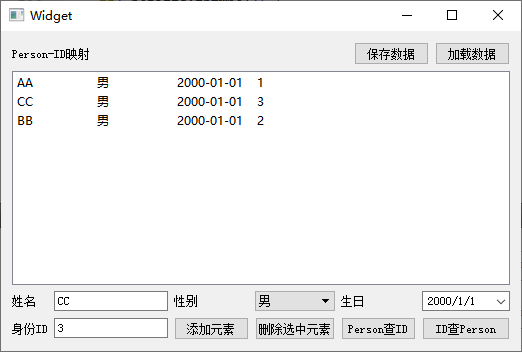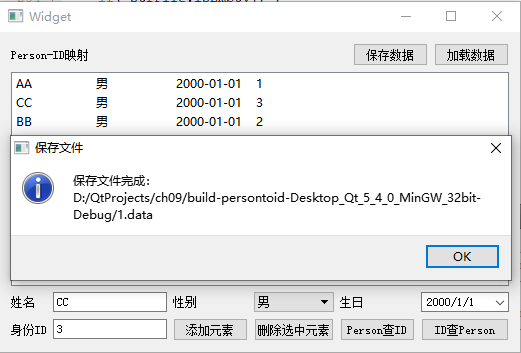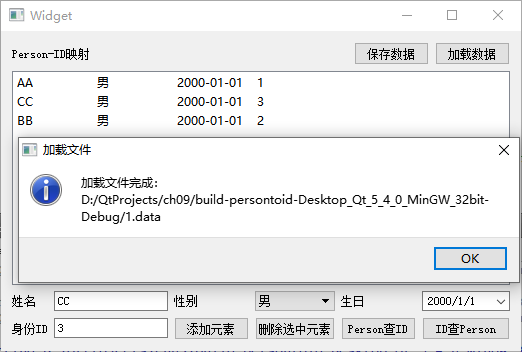9.4.2 多哈希映射 QMultiHash

QMultiHash 是 QHash 的派生类，继承了 QHash 绝大多数的函数，同时也根据一对多映射的特性做了改进，将基类的函数进行了重载。除了基类的函数，QMultiHash 新增了一些函数：
（1）构造函数
QMultiHash()    //默认构造函数
QMultiHash(std::initializer_list<std::pair<Key, T> > list)    //初始化列表构造函数
QMultiHash(const QHash<Key, T> & other)    //复制构造函数

QMultiHash<QString, int> ha1{
{"Alice", 10086},
{"Alice", 10087},
{"Bob", 10010},
{"Bob", 10011}
};
qDebug()<<ha1;

（2）添加函数
QHash<Key, T>::iterator    insert(const Key & key, const T & value)
QMultiHash 使用的迭代器与基类一样，只是有些函数功能有差异，这个 insert() 函数就是最典型的差异。

QMultiHash<QString, int> ha1;
ha1.insert( "AA", 1 );
ha1.insert( "AA", 1 );
ha1.insert( "AA", 1 );
qDebug()<<ha1;

QHash(("AA", 1)("AA", 1)("AA", 1))

（3）删除函数
int    remove(const Key & key, const T & value)
int    remove(const Key & key)

（4）访问和查询函数
count 计数的函数有三个：
int    count(const Key & key, const T & value) const
int    count(const Key & key) const
int    count() const

contains 包含查询有两个函数：
bool    contains(const Key & key, const T & value) const
bool    contains(const Key & key) const

find 查找函数共有 6 个，首先是根据 key-value 对查找元素位置，3个函数：
QHash<Key, T>::const_iterator    constFind(const Key & key, const T & value) const   //只读迭代器查询，Qt风格函数名
QHash<Key, T>::const_iterator    find(const Key & key, const T & value) const    //只读迭代器查询，STL风格函数名
QHash<Key, T>::iterator    find(const Key & key, const T & value)    //读写迭代器查询

QHash<Key, T>::const_iterator    constFind(const Key & key) const    //只读迭代器查询，Qt风格函数名
QHash<Key, T>::const_iterator    find(const Key & key) const    //只读迭代器查询，STL风格函数名
QHash<Key, T>::iterator    find(const Key & key)    //读写迭代器查询
（5）替换和交换函数

QHash<Key, T>::iterator    replace(const Key & key, const T & value)

void    swap(QMultiHash<Key, T> & other)

（6）运算符函数

QMultiHash    operator+(const QMultiHash & other) const
QMultiHash &    operator+=(const QMultiHash & other)
+ 函数返回新的合并后对象， += 函数直接将合并后的对象赋给左值对象。合并后的对象总是合并前两个对象元素个数的总和，而不会检查有没有重复的元素，元素有重复就重复添加。

QHash 和 QMultiHash 二者功能函数的主要区别对比如下表所示：

 功能 QHash 函数 QMultiHash 函数 一对一添加节点 insert(key,value)：无匹配key时直接插入新节点，有匹配key时替换旧节点。 replace(key,value)：无匹配key时直接插入新节点，有匹配key时，替换之前最新插入的同样key节点。 一对多添加节点 insertMulti(key,value)：直接将新节点插入到哈希表，不考虑之前有无同样键值的节点。 insert(key,value)：直接将新节点插入到哈希表，不考虑之前有无同样键值的节点。insertMulti(key,value)函数同基类。 中括号运算符 operator[](key)：根据key读写匹配的节点，如果没有匹配的，自动添加该 key 节点。 没有中括号运算符，对于类似功能，读操作调用 value(key)函数，写操作调用replace(key,value)。 合并两个对象 无 + 和 += 运算符函数。QHash::​unite(other)可以将参数other所有节点添加给自己，类似 += 功能。 通过 + 和 += 运算符函数。 查询函数 count(key)、contains(key)、find(key)、constFind(key)，只需要通过 key 查询节点，因为通常是一对一映射。 不仅有 count(key)、contains(key)、find(key)、constFind(key)，一对多映射查询时还可以根据键值对进行查询count(key,value)、contains(key,value)、find(key,value)、constFind(key,value)。 删除函数 remove(key)，删除匹配 key 的节点。 两个删除函数，remove(key)删除匹配 key 的所有节点，remove(key,value)删除匹配 key-value 的所有节点。

9.4.3 集合 QSet

（1）构造函数
QSet()   //默认构造函数
QSet(std::initializer_list<T> list)    //初始化列表构造函数，C++11特性
QSet(const QSet<T> & other)    //复制构造函数
QSet(QSet && other)     //移动构造函数，C++11特性

QSet<QString> s1{"Alice", "Bob", "Ceil"};
qDebug()<<s1;
QSet<QString> s2 = std::move(s1);
qDebug()<<s1;
qDebug()<<s2;

QSet("Bob", "Alice", "Ceil")
QSet()
QSet("Bob", "Alice", "Ceil")

（2）添加函数
iterator    insert(const T & value)
insert() 会检查集合是否存在相同元素，如果不存在相同的，添加新元素，如果已经有相同的，集合保持不变。与之前的 QMap 和 QHash 类不同的是，集合类没有 insertMulti 函数，因为集合要求元素唯一不重复。

（3）删除函数
bool    remove(const T & value)
remove() 删除匹配数值的元素，如果集合中存在该元素，那么删除了并返回 true，如果没有匹配元素，那么返回 false。

void    clear()

（4）访问和查询函数

bool    contains(const T & value) const

int    count() const
int    size() const

bool    empty() const    //STL风格命名
bool    isEmpty() const   //Qt风格命名

QList<T>    values() const
QList<T>    toList() const

QSet<T>    fromList(const QList<T> & list)
fromList() 静态函数根据参数的列表构造一个新集合返回，新集合中元素值是唯一的，可以快速去重复。

（5）数学集合函数

QSet<T> &    unite(const QSet<T> & other)

QSet<T> &    intersect(const QSet<T> & other)

QSet<T> &    subtract(const QSet<T> & other)

bool    contains(const QSet<T> & other) const

QSet<int> s1{1,2,3};
QSet<int> s2{3,4,5};
s1.unite( s2 );
qDebug()<<s1;

QSet<int> s3{1,2,3};
s3.intersect( s2 );
qDebug()<<s3;

QSet<int> s4{1,2,3};
s4.subtract( s2 );
qDebug()<<s4;

QSet<int> s5{1,2,3,4,5};
qDebug()<<s5.contains( s2 );

QSet(1, 4, 5, 2, 3)
QSet(3)
QSet(1, 2)
true

（6）运算符函数

 运算符函数 举 例 描述 bool operator!=(const QSet & other) const s1 != s2; 两个映射的元素不一样，不等号判断结果为 true。 QSet operator&(const QSet & other) const s3 = s1 & s2; s3 为交集 {3}，s1和s2不变。 QSet & operator&=(const QSet & other) s1 &= s2; s1 为交集 {3}，s2不变。 QSet & operator&=(const T & value) s1 &= 1; s1 和单个数值求交集，s1变为 {1}。 QSet operator+(const QSet & other) const s3 = s1 + s2; s3 为并集{1,2,3,4,5}，s1和s2不变。 QSet & operator+=(const QSet & other) s1 += s2; s1 为并集{1,2,3,4,5}，s2不变。 QSet & operator+=(const T & value) s1 += 8; s1 为合并一个数值的新集合 {1,2,3,8}。 QSet operator-(const QSet & other) const s3 = s1 - s2; s3 为差集{1,2}，s1和s2不变。s3 等同于 s1 - (s1&s2) 。 QSet & operator-=(const QSet & other) s1 -= s2; s1 为差集{1,2}，s2不变。 QSet & operator-=(const T & value) s1 -= 1; s1 为{2,3}，去掉右边单个数值。 QSet & operator<<(const T & value) s1 << 8; 添加一个数值给 s1，s1变成{1,2,3,8}。 QSet & operator=(const QSet & other) s3 = s1; 赋值，s3变成 {1,2,3}，s1不变。 QSet & operator=(QSet && other) s3 = std::move(s1); 移动赋值，s3变成 {1,2,3}，s1变为空集合。 bool operator==(const QSet & other) const s1 == s2; 结果为 false，两个集合不一样。 QSet operator|(const QSet & other) const s3 = s1 | s2; s3 为并集{1,2,3,4,5}，s1和s2不变。 QSet & operator|=(const QSet & other) s1 |= s2; s1 为并集{1,2,3,4,5}，s2不变。 QSet & operator|=(const T & value) s1 |= 8; s1 为合并一个数值的新集合 {1,2,3,8}。

（7）迭代器函数

class    const_iterator  //STL风格只读迭代器
class    iterator            //STL风格读写迭代器
typedef    ConstIterator  //Qt命名风格只读迭代器
typedef    Iterator          //Qt命名风格读写迭代器
STL 风格迭代器使用示范：
QSet<QString>::const_iterator i = set.constBegin();
while (i != set.constEnd()) {
qDebug() << *i;
++i;
}

iterator    begin()     //指向头部的读写迭代器
const_iterator    begin() const            //指向头部的只读迭代器
const_iterator    cbegin() const          //指向头部的只读迭代器
const_iterator    constBegin() const  //指向头部的只读迭代器，Qt风格
iterator    end()       //指向尾部后面假想元素的读写迭代器
const_iterator    end() const             //指向尾部后面假想元素的只读迭代器
const_iterator    cend() const           //指向尾部后面假想元素的只读迭代器
const_iterator    constEnd() const    //指向尾部后面假想元素的只读迭代器，Qt风格

const_iterator    constFind(const T & value) const  //只读迭代器查询，Qt风格命名
const_iterator    find(const T & value) const    //只读迭代器查询，STL风格命名
iterator    find(const T & value)   //读写迭代器查询

iterator    erase(iterator pos)
erase() 函数删除时，不会导致内部哈希表的重构，因此可以在迭代遍历集合元素时，安全地调用 erase() 删除指定位置的元素，各个元素的哈希表位置不会错乱。而 remove() 函数会可能导致内部哈希表重构，所以不要在迭代过程中使用 remove() 函数删除元素，哈希表重构会导致迭代器失效。

（8）其他内容

void    reserve(int size)
reserve() 提前分配 size 大小的空间位置，用于预留内存空间。查询预留空间大小，使用如下函数：
int    capacity() const

void    squeeze()

void    swap(QSet<T> & other)   //交换函数效率很高，并且不会失败
QSet集合类也支持串行化输入输出，前提是存储的数值类型也支持串行化：
QDataStream &    operator<<(QDataStream & out, const QSet<T> & set)
QDataStream &    operator>>(QDataStream & in, QSet<T> & set)

②套件选择里面选择全部套件，点击下一步；
③基类选择 QWidget，点击下一步；
④项目管理不修改，点击完成。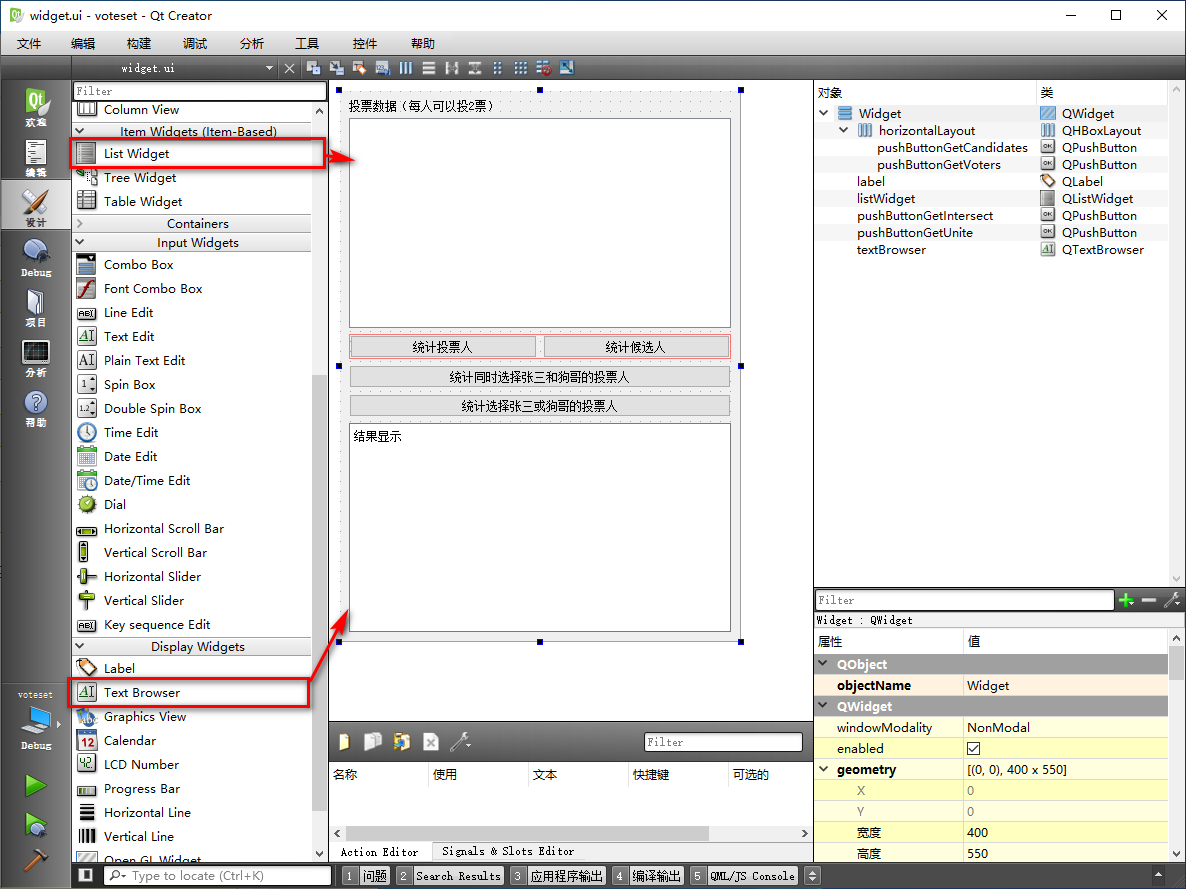#ifndef WIDGET_H
`#define WIDGET_H`

`#include <QWidget>`
`#include <QMultiHash>`
`#include <QSet>`

`namespace Ui {`
`class Widget;`
`}`

`class Widget : public QWidget`
`{`
`    Q_OBJECT`

`public:`
`    explicit Widget(QWidget *parent = 0);`
`    ~Widget();`

`private slots:`
`    void on_pushButtonGetVoters_clicked();`

`    void on_pushButtonGetCandidates_clicked();`

`    void on_pushButtonGetIntersect_clicked();`

`    void on_pushButtonGetUnite_clicked();`

`private:`
`    Ui::Widget *ui;`
`    //保存投票`
`    QMultiHash<QString, QString> m_voteData;`
`    //填充投票，并设置列表框显示`
`    void FillDataAndListWidget();`

`};`

`#endif // WIDGET_H`

#include "widget.h"
`#include "ui_widget.h"`
`#include <QMessageBox>`
`#include <QDebug>`

`Widget::Widget(QWidget *parent) :`
`    QWidget(parent),`
`    ui(new Ui::Widget)`
`{`
`    ui->setupUi(this);`

`    //填充投票，并设置列表框显示`
`    FillDataAndListWidget();`
`}`

`void Widget::FillDataAndListWidget()`
`{`
`    m_voteData.insert( tr("路人甲"), tr("张三") );`
`    m_voteData.insert( tr("路人甲"), tr("狗哥") );`
`    m_voteData.insert( tr("路人乙"), tr("李四") );`
`    m_voteData.insert( tr("路人乙"), tr("狗哥") );`
`    m_voteData.insert( tr("路人丙"), tr("张三") );`
`    m_voteData.insert( tr("路人丙"), tr("狗哥") );`
`    m_voteData.insert( tr("路人丁"), tr("李四") );`
`    m_voteData.insert( tr("路人丁"), tr("狗哥") );`
`    m_voteData.insert( tr("路人戊"), tr("张三") );`
`    m_voteData.insert( tr("路人戊"), tr("狗哥") );`
`    //显示到列表控件`
`    ui->listWidget->clear();`
`    //使用迭代器遍历`
`    QHash<QString, QString>::const_iterator it = m_voteData.constBegin();`
`    while ( it != m_voteData.constEnd() )`
`    {`
`        QString strLine = it.key() + tr("\t投\t") + it.value();`
`        ui->listWidget->addItem( strLine );`
`        ++it;`
`    }`
`}`

`Widget::~Widget()`
`{`
`    delete ui;`
`}`

//统计投票人，不重复的
`void Widget::on_pushButtonGetVoters_clicked()`
`{`
`    //投票人是 keys`
`    QList<QString> listVoters = m_voteData.keys();`
`    //用集合去重复`
`    QSet<QString> uniqueVoters = QSet<QString>::fromList( listVoters );`
`    //可以转回列表`
`    listVoters = uniqueVoters.toList();`
`    //构造信息字符串`
`    int nCount = listVoters.count();`
`    QString strInfo = tr("投票人数：%1 \r\n").arg(nCount);`
`    for(int i=0; i<nCount; i++)`
`    {`
`        strInfo += listVoters[i] + tr("\r\n");`
`    }`
`    //显示`
`    ui->textBrowser->setText( strInfo );`
`}`

QSet<QString>::fromList( listVoters )

//统计候选人，不重复的
`void Widget::on_pushButtonGetCandidates_clicked()`
`{`
`    //候选人是 values`
`    QList<QString> listCandidates = m_voteData.values();`
`    //用集合去重复`
`    QSet<QString> uniqueCandidates = QSet<QString>::fromList( listCandidates );`
`    //转回列表`
`    listCandidates = uniqueCandidates.toList();`
`    //构造信息字符串`
`    int nCount = listCandidates.count();`
`    QString strInfo = tr("候选人数：%1 \r\n").arg(nCount);`
`    for(int i=0; i<nCount; i++)`
`    {`
`        strInfo += listCandidates[i] + tr("\r\n");`
`    }`
`    //显示`
`    ui->textBrowser->setText( strInfo );`
`}`

//统计同时选择张三和狗哥的投票人
`void Widget::on_pushButtonGetIntersect_clicked()`
`{`
`    QSet<QString> voteZhangSan;`
`    QSet<QString> voteGouGe;`
`    //使用迭代器遍历`
`    QHash<QString, QString>::const_iterator it = m_voteData.constBegin();`
`    while ( it != m_voteData.constEnd() )`
`    {`
`        if( it.value() == tr("张三") )`
`        {`
`            voteZhangSan<<it.key();`
`        }`
`        if( it.value() == tr("狗哥") )`
`        {`
`            voteGouGe<<it.key();`
`        }`
`        ++it;`
`    }`
`    //张三的投票人集合，与狗哥的投票人集合 求交集`
`    QSet<QString> sIntersect = voteZhangSan & voteGouGe;`
`    QStringList listIntersect = sIntersect.toList();`
`    //构造信息字符串`
`    QString strInfo = tr("同时选择张三和狗哥的投票人数：%1 \r\n").arg(listIntersect.count());`
`    strInfo += listIntersect.join( "\r\n" );`
`    //显示`
`    ui->textBrowser->setText( strInfo );`
`}`

QList<QString> 转到 QStringList 这个转换过程是自动的，因为 QStringList 类定义了转换的构造函数和赋值函数；

//统计选择张三或狗哥的投票人
`void Widget::on_pushButtonGetUnite_clicked()`
`{`
`    QSet<QString> voteZhangSan;`
`    QSet<QString> voteGouGe;`
`    //使用迭代器遍历`
`    QHash<QString, QString>::const_iterator it = m_voteData.constBegin();`
`    while ( it != m_voteData.constEnd() )`
`    {`
`        if( it.value() == tr("张三") )`
`        {`
`            voteZhangSan<<it.key();`
`        }`
`        if( it.value() == tr("狗哥") )`
`        {`
`            voteGouGe<<it.key();`
`        }`
`        ++it;`
`    }`
`    //张三的投票人集合，与狗哥的投票人集合 求并集`
`    QSet<QString> sUnite = voteZhangSan | voteGouGe;`
`    QStringList listUnite = sUnite.toList();`
`    //构造信息字符串`
`    QString strInfo = tr("选择张三或狗哥的投票人数：%1 \r\n").arg(listUnite.count());`
`    strInfo += listUnite.join( "\r\n" );`
`    //显示`
`    ui->textBrowser->setText( strInfo );`
`}`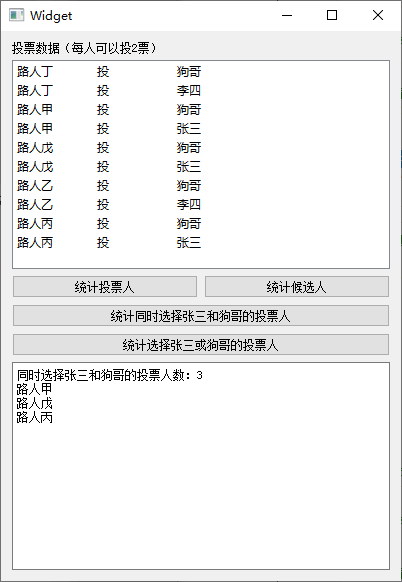9.4.4 关联容器对比

9.3 节和 9.4 节内容介绍了 QMap、QMultiMap、QHash、QMultiHash、QSet 五种容器，前两种是红黑树实现，后三种是哈希表实现。他们的 key 查询和元素插入的时间复杂度如下表所示：

 容器类型 key 查询平均时间 key 查询最坏时间 元素插入平均时间 元素插入最坏时间 QMap / QMultiMap O(log n) O(log n) O(log n) O(log n) QHash / QMultiHash Amort. O(1) O(n) Amort. O(1) O(n) QSet Amort. O(1) O(n) Amort. O(1) O(n)

①项目名称 associativetest，创建路径 D:\QtProjects\ch09，点击下一步；
②套件选择里面选择全部套件，点击下一步；
③基类选择 QWidget，点击下一步；
④项目管理不修改，点击完成。

CONFIG += c++11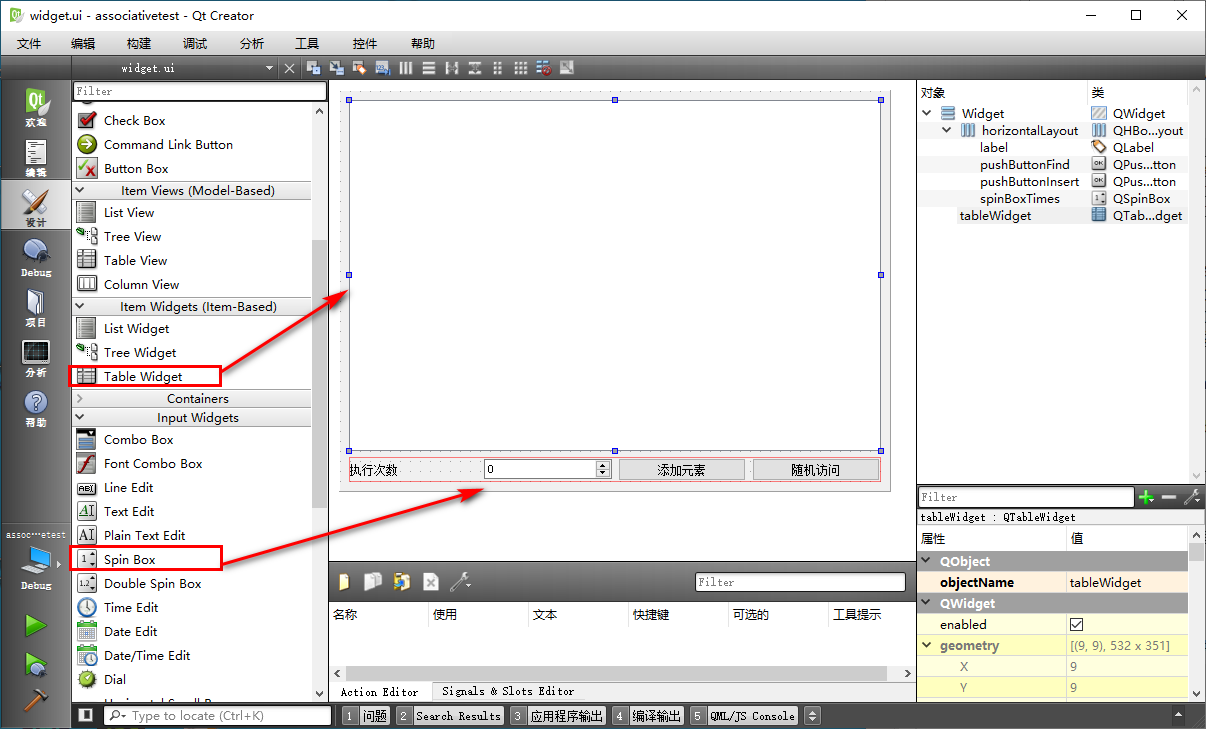#ifndef WIDGET_H
`#define WIDGET_H`

`#include <QWidget>`
`#include <QMap>`
`#include <QHash>`
`#include <QSet>`
`#include <QElapsedTimer> //计时器`
`#include <QLocale> //本地化类，用于打印逗号分隔的数字`

`namespace Ui {`
`class Widget;`
`}`

`class Widget : public QWidget`
`{`
`    Q_OBJECT`

`public:`
`    explicit Widget(QWidget *parent = 0);`
`    ~Widget();`

`private slots:`
`    void on_pushButtonInsert_clicked();`

`    void on_pushButtonFind_clicked();`

`private:`
`    Ui::Widget *ui;`
`    //容器对象`
`    QMap<int, int>  m_map;`
`    QHash<int, int> m_hash;`
`    QSet<int>  m_set;`
`    //耗费计时器`
`    QElapsedTimer m_calcTimer;`
`    //本地化对象，用于打印逗号分隔的数字`
`    QLocale m_locale;`
`};`

`#endif // WIDGET_H`

#include "widget.h"
`#include "ui_widget.h"`
`#include <QMessageBox>`
`#include <QDebug>`
`#include <random>`
`using namespace std;`

`Widget::Widget(QWidget *parent) :`
`    QWidget(parent),`
`    ui(new Ui::Widget)`
`{`
`    ui->setupUi(this);`
`    //窗口标题栏`
`    setWindowTitle( tr("关联容器测试，时间单位：纳秒") );`

`    //设置表格列数`
`    ui->tableWidget->setColumnCount( 4 );`
`    //设置表头`
`    QStringList header;`
`    header<<tr("操作名称")<<tr("QMap")<<tr("QHash")<<tr("QSet");`
`    ui->tableWidget->setHorizontalHeaderLabels( header );`
`    //各列均匀拉伸`
`    ui->tableWidget->horizontalHeader()->setSectionResizeMode( QHeaderView::Stretch );`

`    //设置旋钮编辑框`
`    ui->spinBoxTimes->setSuffix( tr(" 万") ); // 单位万`
`    ui->spinBoxTimes->setRange( 1, 21*10000 ); //21亿上限`
`    //设置英语本地化，每三个数字打逗号`
`    m_locale = QLocale(QLocale::English, QLocale::UnitedStates);`
`    qDebug()<<m_locale.toString( 1234567 );`

`}`

`Widget::~Widget()`
`{`
`    delete ui;`
`}`

//添加元素功能测试
`void Widget::on_pushButtonInsert_clicked()`
`{`
`    //获取测试次数`
`    int nTimes = ui->spinBoxTimes->value() * 10000;`
`    //表格控件添加新行`
`    int nNewRow = ui->tableWidget->rowCount(); //作为新行序号`
`    ui->tableWidget->setRowCount( nNewRow+1 ); //增加一行`
`    //设置第 0 列文本`
`    QString strText = tr("添加元素 %1 万次").arg( ui->spinBoxTimes->value() );`
`    QTableWidgetItem *itemNew = new QTableWidgetItem( strText );`
`    ui->tableWidget->setItem(nNewRow, 0, itemNew);`

`    //C++11特性，定义随机数均匀分布、随机数引擎`
`    uniform_int_distribution<unsigned> ud(0, nTimes);`
`    default_random_engine eg;`
`    //QMap测试`
`    m_map.clear();`
`    qint64 nsUsed = 0; //纳秒计数，耗时包含了随机数产生时间`
`    //开始计时`
`    m_calcTimer.start();`
`    for(int i=0; i<nTimes; i++)`
`    {`
`        int nVal = ud(eg);`
`        m_map.insert(nVal, nVal);`
`    }`
`    nsUsed = m_calcTimer.nsecsElapsed();`
`    //设置文本并新建表格条目`
`    strText = m_locale.toString( nsUsed );`
`    itemNew = new QTableWidgetItem( strText );`
`    ui->tableWidget->setItem( nNewRow, 1, itemNew );`

`    //QHash测试`
`    m_hash.clear(); //清空旧的`
`    nsUsed = 0;`
`    //开始计时`
`    m_calcTimer.start();`
`    for(int i=0; i<nTimes; i++)`
`    {`
`        int nVal = ud(eg);`
`        m_hash.insert(nVal, nVal);`
`    }`
`    nsUsed = m_calcTimer.nsecsElapsed();`
`    //设置文本并新建表格条目`
`    strText = m_locale.toString( nsUsed );`
`    itemNew = new QTableWidgetItem( strText );`
`    ui->tableWidget->setItem( nNewRow, 2, itemNew );`

`    //QSet测试`
`    m_set.clear(); //清空旧的`
`    nsUsed = 0;`
`    //开始计时`
`    m_calcTimer.start();`
`    for(int i=0; i<nTimes; i++)`
`    {`
`        int nVal = ud(eg);`
`        m_set.insert( nVal );`
`    }`
`    nsUsed = m_calcTimer.nsecsElapsed();`
`    //设置文本并新建条目`
`    strText = m_locale.toString( nsUsed );`
`    itemNew = new QTableWidgetItem( strText );`
`    ui->tableWidget->setItem( nNewRow, 3, itemNew );`
`}`

//随机访问功能测试
`void Widget::on_pushButtonFind_clicked()`
`{`
`    //检查是否为空`
`    if( m_map.isEmpty() || m_hash.isEmpty() || m_set.isEmpty() )`
`    {`
`        QMessageBox::warning(this, tr("随机访问"), tr("容器对象为空，请执行添加元素后执行访问！"));`
`        return;`
`    }`
`    //获取测试次数`
`    int nTimes = ui->spinBoxTimes->value() * 10000;`
`    //表格控件增加一行`
`    int nNewRow = ui->tableWidget->rowCount(); //新行的序号`
`    ui->tableWidget->setRowCount( nNewRow+1 ); //加一行`
`    //设置第 0 列文本`
`    QString strText = tr("随机访问 %1 万次").arg( ui->spinBoxTimes->value() );`
`    QTableWidgetItem *itemNew = new QTableWidgetItem( strText );`
`    ui->tableWidget->setItem( nNewRow, 0, itemNew );`

`    //C++11特性，定义随机数均匀分布、随机数引擎`
`    uniform_int_distribution<unsigned> ud(0, nTimes);`
`    default_random_engine eg;`

`    //QMap测试`
`    qint64 nsUsed = 0; //纳秒计数，耗时包含了随机数产生时间`
`    //开始计时`
`    m_calcTimer.start();`
`    for(int i=0; i<nTimes; i++)`
`    {`
`        int nVal = ud(eg);`
`        m_map.find( nVal );`
`    }`
`    nsUsed = m_calcTimer.nsecsElapsed();`
`    //设置文本并新建表格条目`
`    strText = m_locale.toString( nsUsed );`
`    itemNew = new QTableWidgetItem( strText );`
`    ui->tableWidget->setItem( nNewRow, 1, itemNew );`

`    //QHash测试`
`    nsUsed = 0;`
`    //开始计时`
`    m_calcTimer.start();`
`    for(int i=0; i<nTimes; i++)`
`    {`
`        int nVal = ud(eg);`
`        m_hash.find(nVal);`
`    }`
`    nsUsed = m_calcTimer.nsecsElapsed();`
`    //设置文本并新建表格条目`
`    strText = m_locale.toString( nsUsed );`
`    itemNew = new QTableWidgetItem( strText );`
`    ui->tableWidget->setItem( nNewRow, 2, itemNew );`

`    //QSet测试`
`    nsUsed = 0;`
`    //开始计时`
`    m_calcTimer.start();`
`    for(int i=0; i<nTimes; i++)`
`    {`
`        int nVal = ud(eg);`
`        m_set.find( nVal );`
`    }`
`    nsUsed = m_calcTimer.nsecsElapsed();`
`    //设置文本并新建条目`
`    strText = m_locale.toString( nsUsed );`
`    itemNew = new QTableWidgetItem( strText );`
`    ui->tableWidget->setItem( nNewRow, 3, itemNew );`
`}`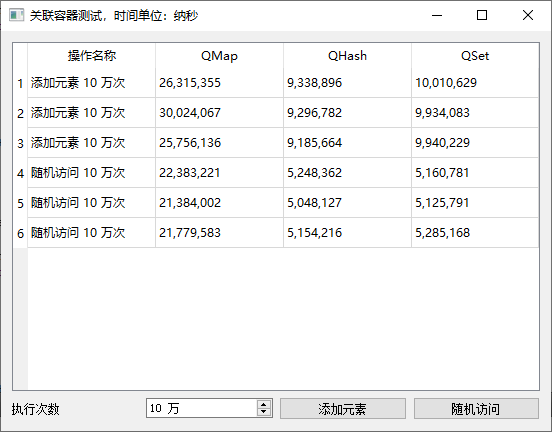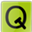练习
① 将 9.3.1 单映射 QMap 小节 nameage 示例中 QMap 内容改用 QHash 实现。
② 将 9.3.2 多映射 QMultiMap 小节的 namephone 例子，用本小节的 QMultiHash 实现。
③ 为 9.4.3 小节 voteset 例子添加一个按钮，实现选举出武林盟主、副盟主的功能，信息显示到文本浏览框。